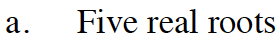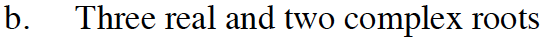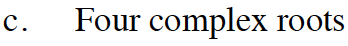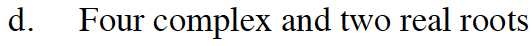### Home > INT3 > Chapter 11 > Lesson 11.2.4 > Problem11-103

11-103.
1. Sketch a graph, y = f(x), that has the number and types of roots described for each situation below. Homework Help ✎

1. Five real roots

2. Three real and two complex roots

3. Four complex roots

4. Four complex and two real rootsSince the degree is an odd number, it will start and end in different directions.
A real root occurs when the polynomial intersects the x-axis, so this graph should intersect the x-axis 5 times.This polynomial has 5 roots, but only 3 x-intercepts.

A possible graph would be a polynomial with 3 x-intercepts plus another bend.The degree of this polynomial is even, so it starts and ends in the same direction.See part (a), (b), and (c).